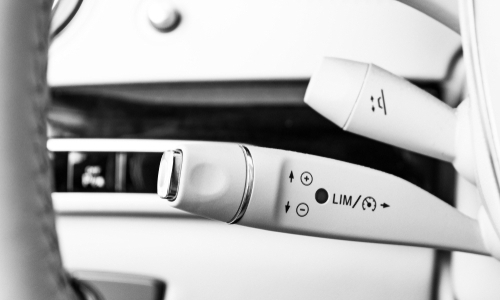# Optimal Tuning of a PI Controller for a Cruise Control SystemNowadays, modern cars incorporate automatic cruise controllers to enhance the driving experience. The main objective of a cruise controller is to maintain car speed despite any external disturbance related to road surfaces or wind profiles. In this example, we will show how to optimally tune a PI controller for a car cruise controller using Collimator.

## Cruise control model

For a cruise control model, we will consider the longitudinal dynamics of a car. The dynamics can be abstracted as the cascade of the engine force dynamics and the car dynamics Figure 1.

The car dynamics can be modeled as a first-order differential equation:

$$mv ̇+bv=F$$

where $$m=1000$$ is the car mass, $$b=50$$ is a dynamical damping factor, $$v$$ is the car speed, and $$F$$ is the engine force. We can build this differential equation in Collimator as a submodel block diagram Figure 2:

The engine dynamics is also modeled as a first-order differential equation:

$$\dot f ̇+λf=u$$

where $$λ=2$$ is the engine traction coefficient, $$u$$ is the the throttle input, and $$f$$ is the generated engine force. The delivered force is amplified by the engine power factor $$μ=100$$

$$F=μf$$

The engine dynamics submodel is shown in Figure 3.

Finally, Figure 4 illustrates the overall cruise control system simulation is implemented in Collimator:

## PI controller tuning for cruise control

Before we start analyzing the model and tuning the controller, we will import some useful libraries:

import numpy as np
import matplotlib.pyplot as plt
import control as ctrl
import collimator as C
from scipy import optimize



From the models we investigated before for the car cruise system, we can abstract the overall model as the linear system block diagram in Figure 5.

Therefore, we will linearize the car dynamics submodel and the engine dynamics submodel to extract the total transfer function of the car cruise system.

my_model = C.load_model('Cruise Control')
car_model = my_model.find_block('car_dynamics')
car_ss  = C.linearize(my_model, car_model).to_state_space()
engine_model = my_model.find_block('engine_dynamics')
engine_ss  = C.linearize(my_model, engine_model).to_state_space()
cruise_tf = ctrl.ss2tf(car_ss) * ctrl.ss2tf(engine_ss)
print(cruise_tf)

     0.2
---------------
s^2 + 2.05s + 1


The open-loop car cruise system is:

$$Gcruise(s)=\frac{V(s)}{U(s)} =\frac{0.2}{s^2+2.05s+0.1}$$

Assuming the PI controller transfer function is $$C(s)$$ and to simply the analysis, we will make use of the following closed loop transfer functions:

1. The output transfer function:
$$\frac{V(s)}{R(s)} =\frac{C(s).G(s)}{1+C(s).G(s) }$$
2. The control action transfer function:
$$\frac{U(s)}{R(s)} =\frac{C(s)}{1+C(s).G(s)}$$
3. The error transfer function:
$$\frac{E(s)}{R(s)} =\frac{1}{1+C(s).G(s)}$$

We will start designing the controller by introducing proportional controller and evaluating some empirical gains:

plt.rcParams["figure.figsize"] = (12,8)
plt.grid(which='both')
plt.xlabel("Time")
plt.ylabel("Speed")
my_model.set_configuration({'stop_time':50.0})
for kp in np.array([1, 5, 10, 50]):
yout = collimator.run_simulation(my_model,{'kp':kp,'ki':0.0}).get_results().to_pandas()
plt.plot(yout['car_dynamics.v'],label="kp = "+str(kp))
plt.legend()


From the output responses above, we might infer that we can use a high proportional gain to have the required controller. However, let’s have a look at the generated control actions first.

plt.rcParams["figure.figsize"] = (12,8)
plt.grid(which='both')
plt.xlabel("Time")
plt.ylabel("Control Action")
my_model.set_configuration({'stop_time':8.0})
for kp in np.array([1, 5, 10, 50]):
u = collimator.run_simulation(my_model,{'kp':kp,'ki':0.0}).get_results().to_pandas()
plt.plot(u['PID_0'],label="kp = "+str(kp))
plt.legend()


From the control action responses, we can see that the controllers with extremely high gains lead to impractical control actions. This means that for this controller to work, we will need a very powerfull engine that might be physically unrealizable. We can further investigate the error responses.

my_model.set_configuration({'stop_time':30.0})
plt.rcParams["figure.figsize"] = (12,8)
plt.grid(which='both')
plt.xlabel("Time")
plt.ylabel("Error")
for kp in np.array([1, 5, 10, 50]):
e = collimator.run_simulation(my_model,{'kp':kp,'ki':0.0}).get_results().to_pandas()
plt.legend()


We can see that increasing the proportional gain reduces the steady state error but might be impractical. Therefore, we will add an integrator to the controller to eliminate the steady error. However, we need to tune two gains $$K_p$$ and $$K_i$$ . Empirical tuning can be tedious.

We will formulate the PI tuning problem as an optimization problem. Tuning controllers for constrained systems is a non-convex optimization problem. This means that we can get stuck at many local minima. Therefore, we need to use a global optimization algorithm such as the simplicial homology global optimization. But first, we need to define an objective function. We can use the following integral performance index as the objective function.

$$J=∫e^2(t)dt+w∫u^2(t)dt$$

The squared error integral reflects a higher penalty on larger errors. Also, a weighted squared control action is added to the objective function to penalize higher control actions. We can define the objective function in the Equation above as:

def cost_fun(x):
s = ctrl.tf('s')
pi_controller = x+x/s
# for e
cruise_closed_e = ctrl.feedback(1,pi_controller*cruise_tf)
T, e = ctrl.step_response(10*cruise_closed_e,T=np.arange(0, 30, 0.1))
# for u
cruise_closed_u = ctrl.feedback(pi_controller,cruise_tf)
T, u = ctrl.step_response(10*cruise_closed_u,T=np.arange(0, 30, 0.1))
return np.square(e).sum() + 0.01*np.square(u).sum()


Then, we run the optimization algorithm to find the optimal gains:

bounds = [(0, 100), (0, 100)] # Bounds for PI gains
results = optimize.shgo(cost_fun, bounds)


results:
fun: 1629.3673958790318
funl: array([1629.36739588])
message: 'Optimization terminated successfully.'
nfev: 131
nit: 2
nlfev: 126
nlhev: O
nljev: 28
success: True
x: array([7.37008947, 0.29039573])
xl: array([[7.37008947, 0.29039573]])



## Car cruise control results

The optimal PI gains are $$K_p=7.37$$ and $$K_i=0.29$$. Now, we investigate the behavior of the obtained controller:

plt.rcParams["figure.figsize"] = (12,8)
plt.grid(which='both')
s = ctrl.tf('s')
pi_controller = results.x+results.x/s
plt.xlabel("Time")
plt.ylabel("Speed")
cruise_closed_y = ctrl.feedback(pi_controller*cruise_tf,1)
T, yout = ctrl.step_response(10*cruise_closed_y,T=np.arange(0, 50, 0.1))
plt.plot(T,yout)
ctrl.step_info(cruise_closed_y)


{'RiseTime': 2.059281027398911,
'SettlingTime’: 3.5694204474914453,
‘SettLinglMin': 0.9032064836020911,
‘SettLinglMax': 1.0,
'overshoot': 0,
'Undershoot': 0,
'Peak': 0.9999845586515097,
'PeakTime’: 177.9218807672659,


plt.rcParams["figure.figsize"] = (12,8)